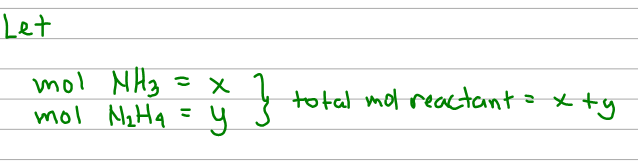# Problem: A mixture of NH3(g) and N2H4(g) is placed in a sealed container at 320 K . The total pressure is 0.49 atm . The container is heated to 1200 K at which time both substances decompose completely according to the equations 2 NH3(g) → N2(g) + 3 H2(g); N2H4(g) → N2(g) + 2 H2(g). After decomposition is complete the total pressure at 1200 K is found to be 4.5 atm.Find the percent (by volume) of N2H4(g) in the original mixture. (Assume two significant figures for the temperature.)

🤓 Based on our data, we think this question is relevant for Professor Tang's class at USF.

###### FREE Expert Solution

First, we set variables for the moles of each reactant:We can add them up to get the total initial moles reactant.

Next, we use these variables to express the amount of product by doing a mole to mole comparison using the coefficients of the balanced reaction. The mole products are just the sum of its coefficient per reaction.###### Problem Details

A mixture of NH3(g) and N2H4(g) is placed in a sealed container at 320 K . The total pressure is 0.49 atm . The container is heated to 1200 K at which time both substances decompose completely according to the equations 2 NH3(g) → N2(g) + 3 H2(g); N2H4(g) → N2(g) + 2 H2(g). After decomposition is complete the total pressure at 1200 K is found to be 4.5 atm.

Find the percent (by volume) of N2H4(g) in the original mixture. (Assume two significant figures for the temperature.)